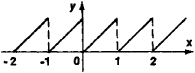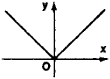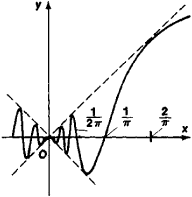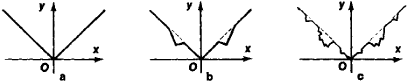# continuous function

(redirected from Noncontinuous function)

## continuous function

[kən¦tin·yə·wəs ′fəŋk·shən]
(mathematics)
A function which is continuous at each point of its domain. Also known as continuous transformation.
McGraw-Hill Dictionary of Scientific & Technical Terms, 6E, Copyright © 2003 by The McGraw-Hill Companies, Inc.
The following article is from The Great Soviet Encyclopedia (1979). It might be outdated or ideologically biased.

## Continuous Function

a function that acquires infinitely small increments for infinitely small increments of the argument. A single-valued function f(x) is continuous for a value x0 of the argument if for all values x of the argument differing by a sufficiently small amount from f(x0), the values of f(x) differ by arbitrarily small amounts from f(x0). More precisely, a function f(x) is said to be continuous for a value x0 of the argument (or, as is usually said, at a point xo) if for an arbitrary ∈ > 0 it is possible to find a δ > 0 such that \f(x) —f(xo)\< ∈ when \x —xo \ < δ. This definition is equivalent to the statement that a function f(x) is continuous at a point x0 if the value of f(x) approaches the limit f(x0) as x approaches xo If all the conditions in the definition of a continuous function hold only when x ≥ x0 (x ≤ xo), then the function is said to be continuous from the right (left) at x0. A function f(x) is said to be continuous on an interval [a, b] if it is continuous at each point x, for a < x < b, and, moreover, it is continuous from the right at a and from the left at b.

The opposite of a continuous function is a discontinuous function. The same function can be continuous for some values of the argument and discontinuous for others. Thus, the fractional part of a number* [usually denoted by (x), for example, (4/3) = 1/3; (Π) = 0.14159 . . . ; and (2) = 0] is a function that is discontinuous for all integral values, continuous for all other values (Figure 1), and continuous from the right at integral points.

Polynomials, the sine (y = sin x), the cosine (y = cos x), and exponential functions (y = ax, where a is a positive number)Figure 1

are the simplest functions of a variable x that are continuous for all values of x. The sum, difference, and product of continuous functions again yield continuous functions. The quotient of two continuous functions is also a continuous function, except for those values of x for which the denominator vanishes (since at these points the given quotient is not defined). For example, tan x = sin x/cos x is a continuous function for all values of x other than odd multiples of Π/2 (for such values, cos x vanishes).

Continuous functions have many important properties that account for the great importance of these functions in mathematics and applications. One of the most important properties is expressed in the theorem that states that for every function continuous on a closed interval [a, b], it is possible to find a polynomial whose values differ on this interval from the values of the function by less than any arbitrarily small preassigned number (Weierstrass’ theorem). The converse theorem, which states that every function that can be replaced on an interval to within an arbitrary degree of precision by a polynomial is continuous on that interval, is also true.Figure 2

A function continuous on a closed interval is bounded on that interval and attains its greatest and least value. Moreover, on this interval it takes on all values between its least and greatest values. Functions continuous on a closed interval are uniformly continuous (on that interval). Every function continuous on an interval is integrable on that interval, that is, it is a derivative of a continuous function. However, not every continuous function has a derivative. Geometrically this means that the graph of a continuous function does not necessarily have a definite direction (tangent) at every point. For example, this may happen because the graph has a corner (Figure 2, the function y = ǀxǀ) or because in every neighborhood of O it oscillates infinitely many times between two intersecting lines [Figure 3, the function y = x sin (ǀl/xǀ) when x ≠ 0 and y = 0 when x = 0].Figure 3

There exist continuous functions nowhere differentiable (the first example of such a function was found by B. Bolzano). The graph of such a function is given in Figure 4, which depicts the first stages of the construction, consisting in the indefinite replacement of the middle third of each line segment by a broken line made up of two segments: the ratio of the lengths is selected such that in the limit we obtain a continuous function.

A function F (x, y, z, .. . ) of several variables defined in some neighborhood of a point (x0, y0, z0, .. .) is said to be continuous at this point if for any ∈ < 0 we can find a δ < 0 such thatFigure 4

if the inequalities ǀx—xoǀ < δ, \y —yoǀ < δ, ǀz —zoǀ < δ, . . . are simultaneously satisfied, then

ǀF(x, y,z, . . .)—F(x0, y0, z0, . . . )ǀ< ∈

Such a function will be continuous in each argument separately (if definite values are assigned to the remaining arguments). The converse, however, is false: a function F(x, y, z, .. . ) continuous in each argument separately may not be a continuous function of these arguments. The simplest example is given by the function F(x, y), which is equal to xy/(x2 + y2) if x2 + y2 ≠ 0 and to zero when x = y = 0. This function is continuous in x for any fixed value of y and in y for any fixed value of x. In particular, it is continuous in x when y = 0 and in y when x = 0. However, if we assume, for example, that y = x ≠ 0, then the value of the function will remain equal to x2/(x2 + x2) = 1/2. It follows that for < ½ it is impossible to find δ such that if ǀxǀ < δ and ǀyǀ < δ hold simultaneously, then ǀxy/(x2 + y2 < ∈. All the fundamental theorems that hold for continuous functions of one variable extend to continuous functions of several variables.

### REFERENCES

Khinchin, A. la. Kratkii kurs matematicheskogo analiza. Moscow, 1953.
Kudriavtsev, L. D. Matematicheskii analiz, vol. 1. Moscow, 1970.

## continuous function

A function f : D -> E, where D and E are cpos, is continuous if it is monotonic and

f (lub Z) = lub { f z | z in Z }

for all directed sets Z in D. In other words, the image of the lub is the lub of any directed image.

All additive functions (functions which preserve all lubs) are continuous. A continuous function has a least fixed point if its domain has a least element, bottom (i.e. it is a cpo or a "pointed cpo" depending on your definition of a cpo). The least fixed point is

fix f = lub f^n bottom | n = 0..infinity